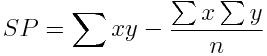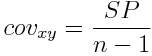The covariance is a measure of how much two numerical variables "vary together." If the two variables "get big together," the covariance will have a high positive value. If one variable gets big while the other gets small, the covariance will have a large negative value. A covariance near zero indicates that the two variables are not related, i.e., that they do not covary. To calculate the covariance, first calculate the sum of products, SP:Then use the sum of products to calculate the covariance.Notice the analogous structure between the variance formula and the covariance formula. This will make the covariance formula easy to remember. Covariance is sort of like the "variance of two variables at once." The covariance is used in statistical calculations, most commonly to calculate the correlation coefficient (following). Return To Main Page